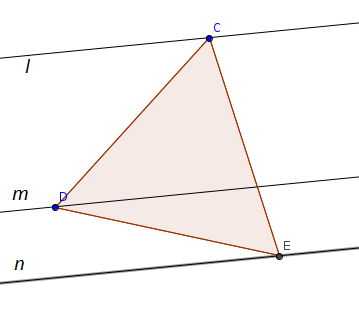# Constrained Triangle

Geometry Level 5We have three parallel lines $l, m$ and $n$ and an equilateral triangle $CDE$ with its vertices on these lines as shown in figure.

The distance between the lines $l$ and $m$ is 5 and between $m$ and $n$ is 2.

The side length of triangle can be expressed as $A \sqrt{B}$, where $A$ and $B$ are positive integers with $B$ square-free. Find $A + B$.

×

Problem Loading...

Note Loading...

Set Loading...